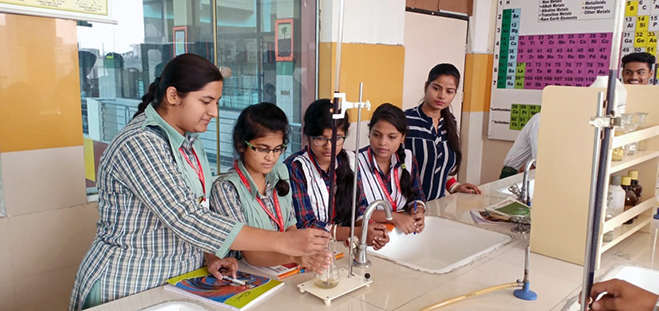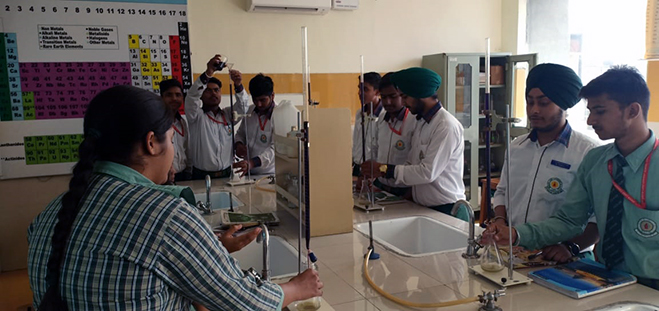08872007170, 08872007171

# Facilities

 Computer LabIn Green Land School there is facility of computer Lab to all its students. Computer Lab is beneficial to students in terms of learning the basic concepts of computer’s and their operation. The students can implement the theoretical concepts being taught in class in to practical.

 Computer LabScience Lab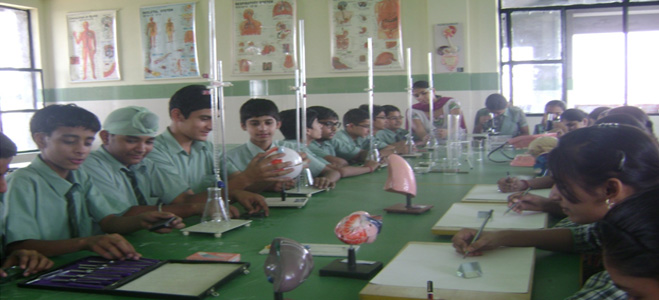Green Land School Civil City provides the facility of Science Lab to students. Science Lab is well equipped with the instruments where the students can perform the experiments. The lab is spacious and well ventilated. Lab is equipped with first-aid to handle any accidental situation.

 Science Lab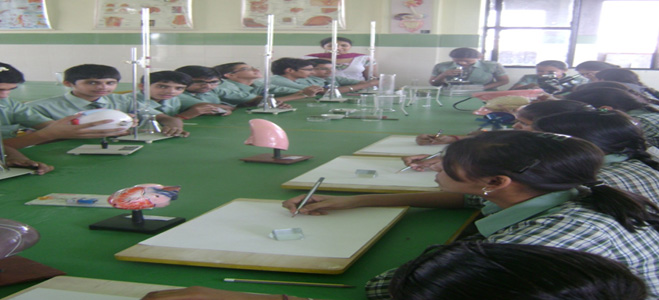LibraryGreen Land School Civil City provides the facility of Library to its students. The library has capacity to accommodate many students at a time. The library contains thousands of books related to student’s curriculum as well as the current affairs. Books in library help the students in increasing their general knowledge and keeping up to date with current affairs.

 Toy Room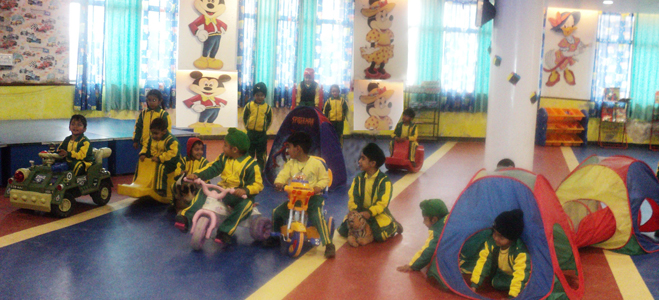Green Land School Civil City provides the facility of Toy Room to its students.

 English Language Lab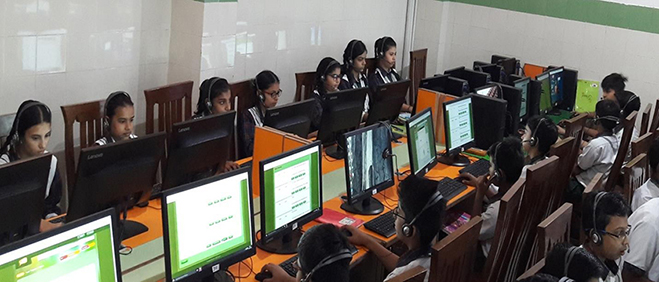Resource Centre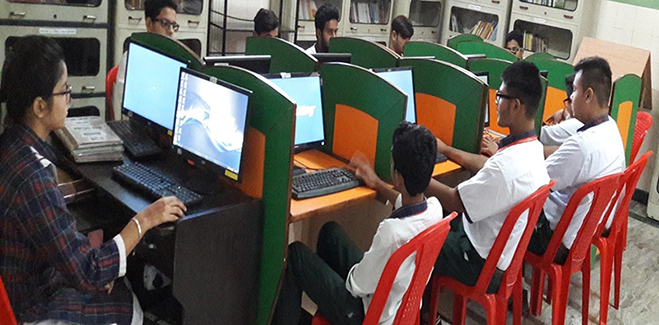Maths Lab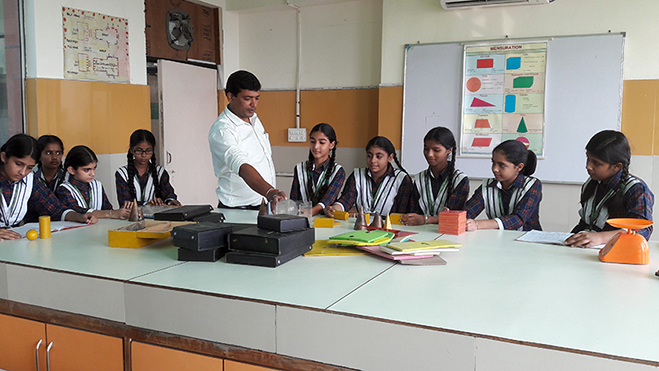Maths Lab at Green Land School provides an unique opportunity to students to understand, internalize discover & verify the basic mathematical & geometrical concepts .Maths Lab is helpful in building interest & confidence in students for learning the mathematical concepts in easiest way.

Advantages of Maths Lab to Students:
1. The Maths Lab helps the students to verify or discover several geometrical properties & facts.
2. Using tools like ‘The geometers sketchpad’ the students will be able to create an object & explore its mathematical properties through and exiting visual process, a process that would help in building a strong foundation in Euclidean & non – Euclidean geometry.
3. Maths lab provides greater scope for individual participation in the process of learning.
4. Maths Lab helps in developing the habit of verifications in the students. They accept mathematical truths only after testing their validity practically.

 Biology Lab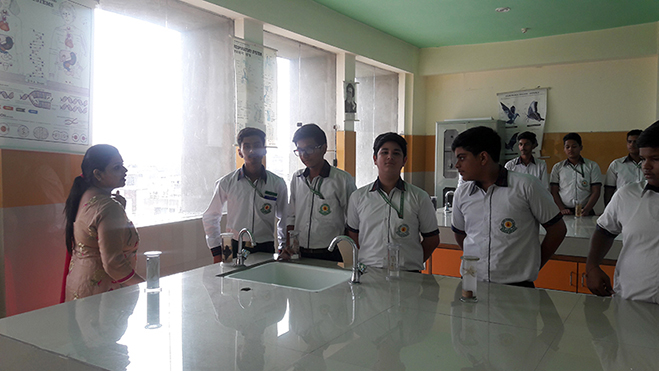MI RoomPhysics Lab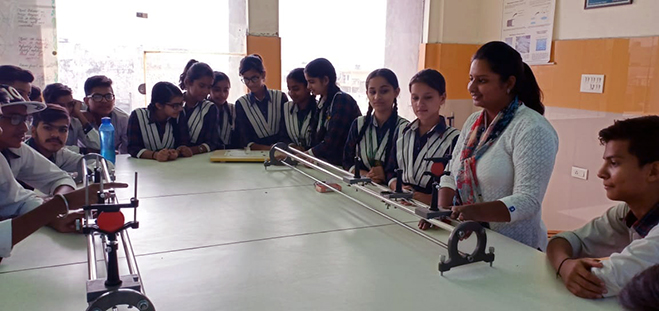Chemistry Lab